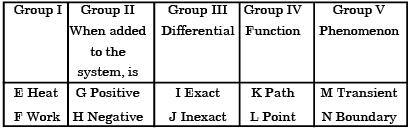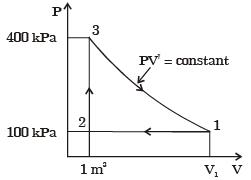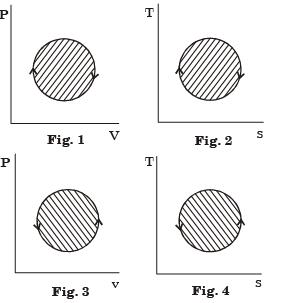Courses

# Past Year Questions: Thermodynamic System And Processes Notes | EduRev

## Mechanical Engineering : Past Year Questions: Thermodynamic System And Processes Notes | EduRev

The document Past Year Questions: Thermodynamic System And Processes Notes | EduRev is a part of the Mechanical Engineering Course Thermodynamics.
All you need of Mechanical Engineering at this link: Mechanical Engineering

Question 1:Which of the following statements are TRUE with respect to heat and work?
(i) They are boundary phenomena
(ii) They are exact differentials
(iii) They are path functions

[2016, Set-1]

Question 2:Heat and work are



Question 3:Match items from groups I, II, III, IV and V.Question 4:The specific heats of an ideal gas depend on its



Question 5:The definition of 1 K as per the internationally accepted temperature scale is



Question 6:An insulated rigid vessel contains a mixture of fuel and air. The mixture is ignited by a minute spark. The contents of the vessel experience



Question 7:During a non-flow thermodynamic process (1-2) executed by a perfect gas, the heat interaction is equal to the work interaction (Q12 = W12) when the process is

[2019 , Set -1]

Question 8:The volume and temperature of air (assumed to be an ideal gas) in a closed vessel is 2.87 m3 and 300 K, respectively. The gauge pressure indicated by a manometer fitted to the wall of the vessel is 0.5 bar. If the gas constant of air is R = 287 J/kgK and the atmospheric pressure is 1 bar, the mass of air (in kg) in the vessel is

[2017, Set-2]

Question 9:A certain amount of an ideal gas is initially at a pressure p1 and temperature T1 First, it undergoes a constant pressure process 1-2 such that T2 = 3T1/4. Then, it undergoes a constant volume process 2-3 such that T3 = T1/2. The ratio of the final volume to the initial volume of the ideal gas is

[2014, Set-3]

Question 10:If the specific heats of the working fluid are constant and the value of specific heat ratio γ is 1.4, the thermal efficiency (%) of the cycle is



Question 11:A thermodynamic cycle with an ideal gas as working fluid is shown below.The above cycle is represented on T-s plane by



Question 12:A reversible thermodynamic cycle containing only three processes and producing work is to be constructed. The constraints are :
(i) there must be one isothermal process,
(ii) there must be one isentropic process,
(iii) the maximum and minimum cycle pressures and the clearance volume are fixed, and
(iv) polytropic processes are not allowed. Then the number of possible cycles are



Question 13:The following four figures have been drawn to represent a fictitious thermodynamic cycle, on thep-v and T-s planes.According to the first law of thermodynamics, equal areas are enclosed by



*Multiple options can be correct
Question 14:An isolated thermodynamic system executes a process. Choose the correct statement (s) from the following



Offer running on EduRev: Apply code STAYHOME200 to get INR 200 off on our premium plan EduRev Infinity!

## Thermodynamics

56 videos|92 docs|33 tests

,

,

,

,

,

,

,

,

,

,

,

,

,

,

,

,

,

,

,

,

,

;